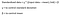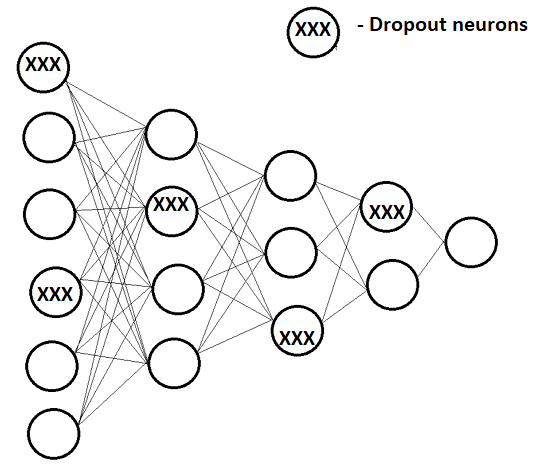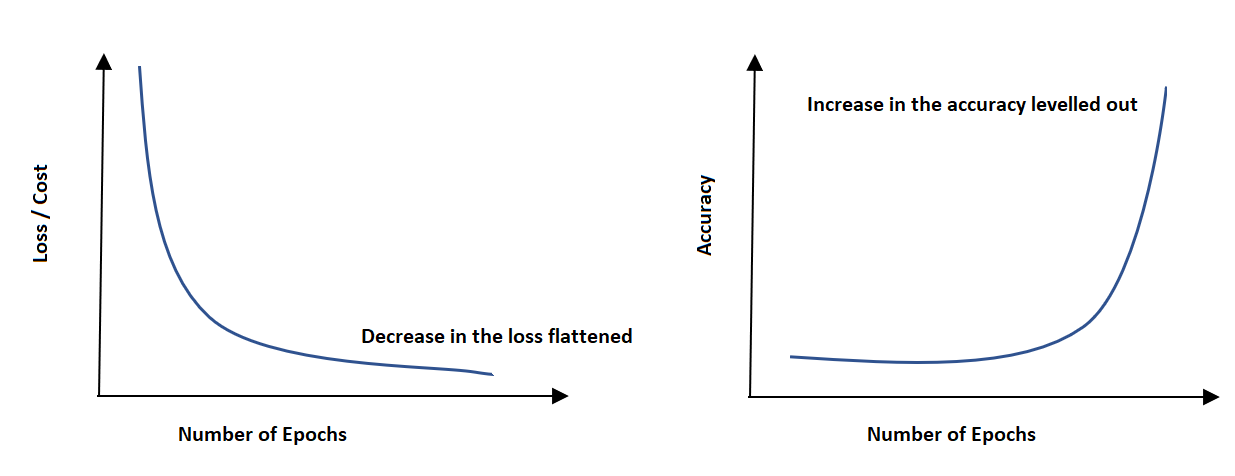# Day 47(DL) — Batch Normalisation, Drop out & Early Stopping

This post is a continuation of Day 45 article that discusses different optimization techniques. The next set of procedures in the row is Batch Normalisation, Drop out and Early Stopping.

Batch Normalization: In general normalising the inputs speed up the learning process(as the steps taken by each feature will be in a circular path rather than an elongated one). In the case of deep neural networks, the same technique applies but on all the layers.

• For instance, let’s say an FNN is created using 10 hidden layers. Every layer learns something new which makes the distribution of the corresponding outputs to vary. As a result, the gaussian distribution is lost when the initially standardised data is passed through the network. This is also termed as internal covariance shift. So, in order to maintain the distribution(mean=0, variance =1) throughout, the batch normalization is applied to each layer in the deep neural network.
• We’ve already discussed that mini-batches are one of the effective ways to train the network. Thus the normalisation for every layer is applied on the batch instead of the complete data. As any training samples fall out of the batch will not contribute to the learning for that iteration.
• Now comes the question of where exactly should we perform the batch normalisation. Is it before or after the activation function?. And the answer is before the activation functions(relu, tanh or sigmoid). The intuitive explanation could be, take the case of tanh the data variation happens between -1 and 1 after that it becomes flat. So it is better to compress the data before passing on to the activation. This would result in faster learning.
• So how do we do the batch normalisation during testing as the mean and variance are computed for the mini-batches?. One constructive way is to take the moving averages of the statistical parameters across the batches. It will become crystal clear when we implement the technique from scratch (in the upcoming articles).
• Can we make the mean and variance flexible(learnable) instead of a constant evaluation only from the data fed in?. Yes, it is possible by introducing two more learnable parameters beta and gamma. They are similar to weights and bias but carry a different meaning. ‘beta’ corresponds to mean and ‘gamma’ for standard deviation. So the formula for the standardization including ‘beta’ and ‘gamma’ is as follows,The formula for standardised data
• One of the implicit advantages of the batch normalisation is it acts as a regularizer. Since we are introducing a minimal noise to the network by performing standardization of the actual data, it prevents the network from overfitting. We know that high variance is the outcome of the model absorbing the training patterns as such, but because of the normalisation noise, the high variance is reduced to a greater extent.

Drop out: As the network becomes too deep, it would end up creating a highly complex function. Recollecting the bias-variance trade-off graph, as the complexity of the model increases, it would result in overfitting(high variance).Fig 1— shows random dropout of neurons in each layer
• Another impressive technique is a dropout. During the training process, the neurons in every layer are randomly dropped out. Subsequently, the overall function becomes less complicated. Thus helps in alleviating the high variance.
• Moreover randomly dropping out the neurons assists the learning process to be equally spread across all the nodes rather than depending on only a few(features).
• Unlike batch normalization, we do not apply dropout during testing as the trained(learnt) model should not be altered during the testing phase.

Early Stopping: We know the overfitting happens when the model absorbs minute details from the data apart from the intended generic ones. One can observe that as the number of epochs increases, the variation in the output accuracy and the reduction in the cost will become minimal. Let’s picturise with a graph,Fig2 — shows epochs Vs cost / accuracy

We can stop the model from further training, once the difference in the accuracies or loss becomes insignificant. This is known as ‘Early Stopping’.

Recommended videos:

https://www.youtube.com/watch?v=ARq74QuavAo&list=RDCMUCcIXc5mJsHVYTZR1maL5l9w&index=2

https://www.youtube.com/watch?v=nUUqwaxLnWs

AI Enthusiast | Blogger✍

## More from Nandhini N

AI Enthusiast | Blogger✍